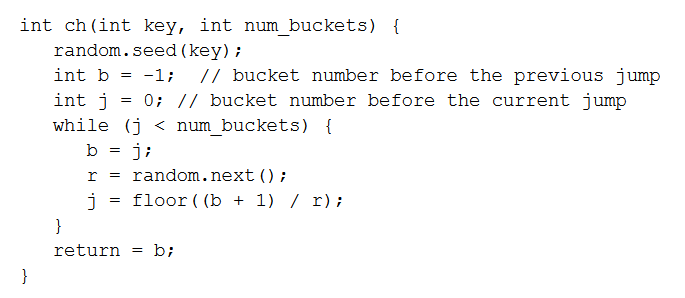# Jump Consistent Hash Algorithm

Contents

One of my colleagues introduced me a new consistent hash algorithm: jump consistent hash. It is a concise algorithm, just in 5 line of code. The paper can be found here:  A Fast, Minimal Memory, Consistent Hash Algorithm. The proofs in the paper are not that detailed, so in this blog, I will try to provide a more formal way to help you understand it and build it from scratch.

## Consistent Hash Algorithm in Distributed System

A hash algorithm has to satisfy some properties to be used in a production environment. The most important two of them are:

1. monotonicity: data can only be transferred from old nodes to new added nodes
2. balance: each tuple is dispatched with the same probability to each segment

For more details, please refer this paper:  Consistent Hashing and Random Trees.

## Scan Algorithm with complexity O(N)

Let’s first focus on the O(n) complexity algorithm, the pseudo-code is shown below(I copy from the paper):

int ch(int key, int N)
{
random.seed(key);
int b = 0; int i;
for (i = 1; i &amp;amp;amp;amp;lt; N; i++)
if (random.next() &amp;amp;amp;amp;lt; (1.0 / (i+1)))
b = i;
return b;
}

Let me re-state this algorithm to make it clearer:

use the data(key) to initialize a pseudo-random generator(This is the most beautiful idea of the paper in my opinion since it is deterministic for each tuple and also we could use probability tools to analyze it)
since the  pseudo-random generator has been initialized, then the output sequence is determined, let’s say it is R_i, 0 \le I \le N-1
the algorithm is to scan R_i to find a index where the following event happens: R_i < \frac{1}{i+1}. We are only interested in the largest index where the event happens. And that is the target Node we return.

Before we analyze it let’s summarize some key points:

• In a cluster of N nodes, for each tuple we should always return the same result to make it work correctly.
• We want to use probability tools to analyze it to make sure that data distribution is balanced.
• To feed each tuple to the Pseudo-Random generator is the highlight of the algorithm.

I believe this model is sort of similar to the popular question in coder interview:

You are given a random number generator which outputs a float number between (0, 1) uniformly. And you are also given an array object arr. This array object arr has only one public method ‘getNext’, the first time it’s called it outputs the first element in the array, the second time it’s called it outputs the second element… When there are no more elements, it outputs NIL.

Design an algorithm that sample one element in arr with equivalent probability.

I solve the problem in the interview of Internship of Alibaba-Inc in 2013. It just came to my mind when I read the code in the jump consistent hash paper.

Code is cheap, show me your proof.

### Proof of Balance

Proof of monotoncity is so easy that I skip it here. Let’s do some warm up by proving balance.

Lemma

\forall key, \forall 0 \le i \le N-1, p(ch(key, N) = i) = \frac{1}{N}.

Proof

We take pseudo random as real random to analyze the model approximately.

p(ch(key, N) = i) =  p(R_i < \frac{1}{i+1} \land R_j > \frac{1}{j+1} \forall i< j < N) = \frac{1}{i+1} \prod_{i+1}^{N-1}\frac{j}{j+1} = \frac{1}{N}

## Improve performance of linear scan

The time complexity of the above algorithm is obviously O(N). It is a linear time complexity algorithm for the cluster size N. The hash function will be called at each time we access the data. O(N) time complexity to access is nightmare.

How can we improve the performance so that it is a practical algorithm?

A fact: for each tuple in a node, the probability for it jumps to the new node has to be \frac{1}{N+1}. That means only little data will change their place. In other words, the sequence in the above algorithm R_i, the event R_i < \frac{1}{I+1} will only happen at few indexes.

Can we model these jump indexes? Imagine that they are a Markov Chain. Can we model the jump process? Then we might get a much faster algorithm.

It can be computed by recurrence:

p(i) = \sum_{j=0}^{i-1}p(j)p(j\_jump\_to\_i|i). We know that p(i)=\frac{1}{i+1}, so that we can design the jump probability to p(j \rightarrow i) = \frac{j+1}{i(i+1)} to make the equation correct(This step is tricky).

So, to model the event using a random number,

p(j \rightarrow i) = \frac{j+1}{i(i+1)}  = p(\frac{j+1}{i+1} < r \le \frac{j+1}{i}) = p(i = \lfloor \frac{j+1}{r} \rfloor)

That’s how we can simulate the jump process using the code in the paper. We first get the random number, then we try to guess the next jump.Algorithm in the paper

### Time complexity

Time complexity analysis is to ask the question:

In these events, R_i < \frac{1}{1+i}, 0 \le i < N, there k of them happen. What is the expectation of k.

By introducing an indicator random variable, we can solve the problem.

X_i = 1 if R_i < \frac{1}{i+1}, so k = \sum_{i=0}^{N-1}X_i .

Then E(k) = \sum_{i=0}^{N-1}E(X_i) = \sum_{i=0}^{N-1}\frac{1}{i+1}. This is Harmonic series. So the time complexity is O(log(N)).

## 6 thoughts on “Jump Consistent Hash Algorithm”

1.Keshav Goel on said:

Here is part i cannot understand in proof of balance is –
how P(Ri < 1/(i+1)) = 1/i+1 ?

Since Ri is random number generator, so by this rule shouldn't it be i/N-1 ?

•kainwenlv on said:

•Keshav Goel on said: